# 可视化拖拽组件库一些技术要点原理分析（二）

1. 编辑器
2. 自定义组件
3. 拖拽
4. 删除组件、调整图层层级
5. 放大缩小
6. 撤消、重做
7. 组件属性设置
8. 吸附
9. 预览、保存代码
10. 绑定事件
11. 绑定动画
12. 导入 PSD
13. 手机模式

• 拖拽旋转
• 复制粘贴剪切
• 数据交互
• 发布

## 14. 拖拽旋转

1. 不支持拖拽旋转。
2. 旋转后的放大缩小不正确。
3. 旋转后的自动吸附不正确。
4. 旋转后八个可伸缩点的光标不正确。

### 拖拽旋转

Math.atan2() 返回从原点(0,0)到(x,y)点的线段与x轴正方向之间的平面角度(弧度值)，也就是Math.atan2(y,x)。Math.atan2(y,x)中的y和x都是相对于圆点(0,0)的距离。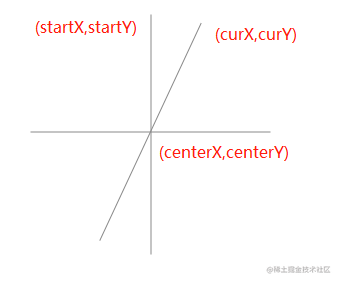``````const startY = e.clientY
const startX = e.clientX

``````// 获取组件中心点位置
const rect = this.\$el.getBoundingClientRect()
const centerX = rect.left + rect.width / 2
const centerY = rect.top + rect.height / 2

``````const curX = moveEvent.clientX
const curY = moveEvent.clientY

``````// 旋转前的角度
const rotateDegreeBefore = Math.atan2(startY - centerY, startX - centerX) / (Math.PI / 180)
// 旋转后的角度
const rotateDegreeAfter = Math.atan2(curY - centerY, curX - centerX) / (Math.PI / 180)
// 获取旋转的角度值， startRotate 为初始角度值
pos.rotate = startRotate + rotateDegreeAfter - rotateDegreeBefore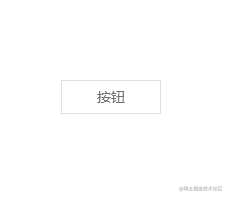### 放大缩小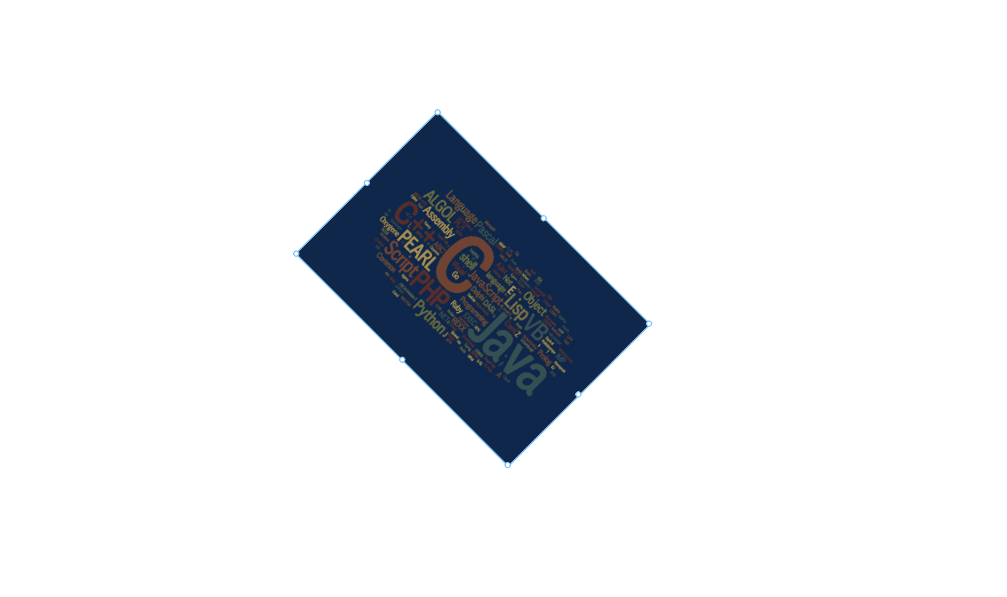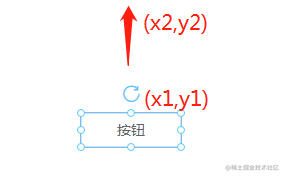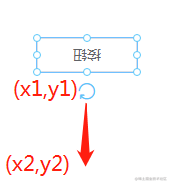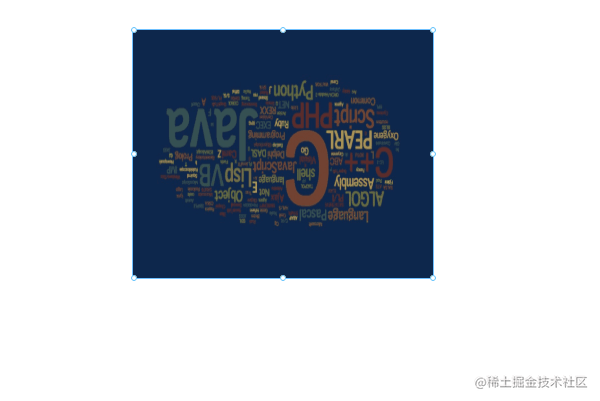#### 解决方案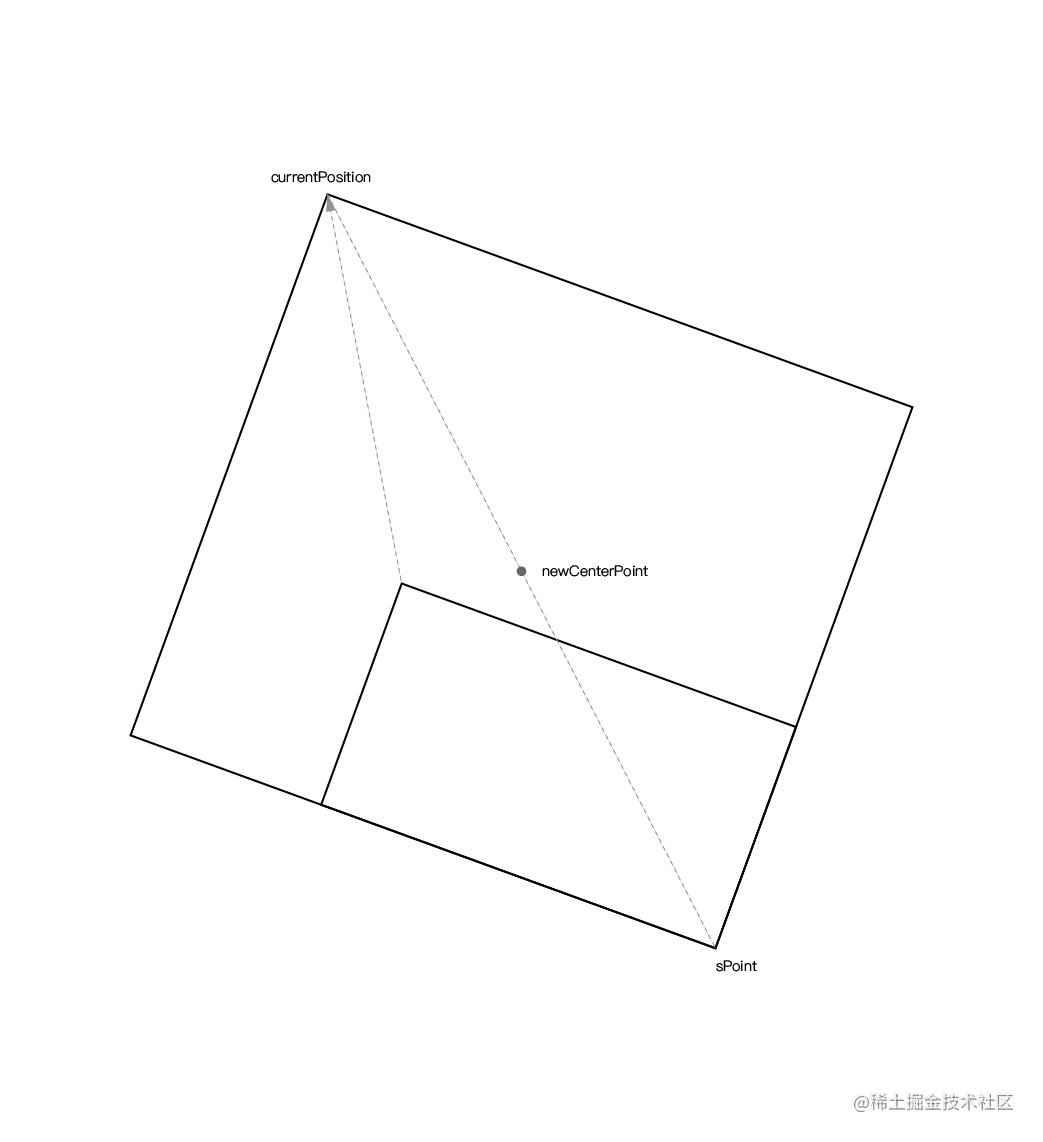``````const center = {
x: style.left + style.width / 2,
y: style.top + style.height / 2,
}

``````// 获取画布位移信息
const editorRectInfo = document.querySelector('#editor').getBoundingClientRect()

// 当前点击坐标
const curPoint = {
x: e.clientX - editorRectInfo.left,
y: e.clientY - editorRectInfo.top,
}

// 获取对称点的坐标
const symmetricPoint = {
x: center.x - (curPoint.x - center.x),
y: center.y - (curPoint.y - center.y),
}

``````const curPositon = {
x: moveEvent.clientX - editorRectInfo.left,
y: moveEvent.clientY - editorRectInfo.top,
}

const newCenterPoint = getCenterPoint(curPositon, symmetricPoint)

// 求两点之间的中点坐标
function getCenterPoint(p1, p2) {
return {
x: p1.x + ((p2.x - p1.x) / 2),
y: p1.y + ((p2.y - p1.y) / 2),
}
}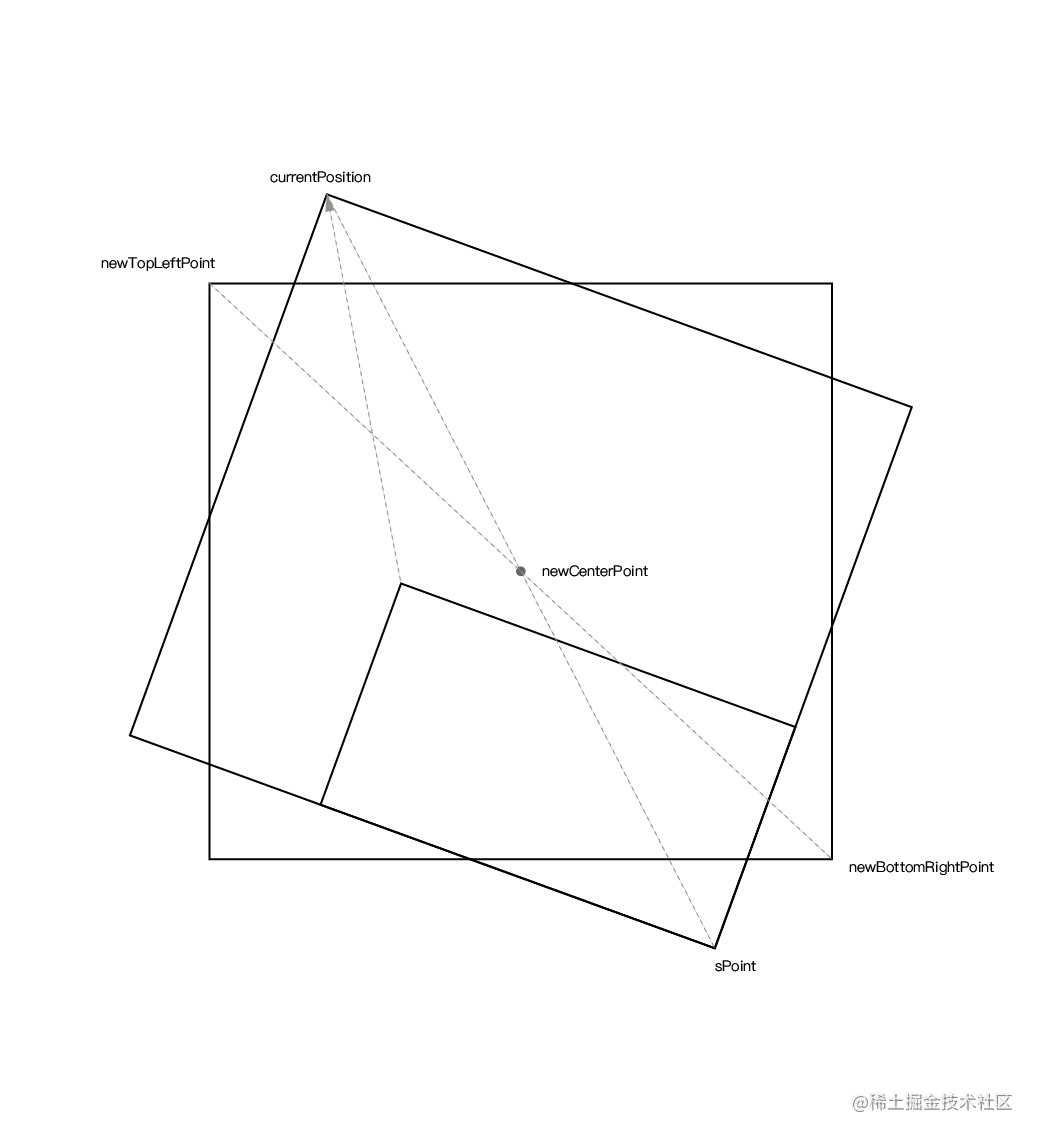``````/**
* 计算根据圆心旋转后的点的坐标
* @param   {Object}  point  旋转前的点坐标
* @param   {Object}  center 旋转中心
* @param   {Number}  rotate 旋转的角度
* @return  {Object}         旋转后的坐标
*/
export function calculateRotatedPointCoordinate(point, center, rotate) {
/**
* 旋转公式：
*  点a(x, y)
*  旋转中心c(x, y)
*  旋转后点n(x, y)
*  旋转角度θ                tan ??
* nx = cosθ * (ax - cx) - sinθ * (ay - cy) + cx
* ny = sinθ * (ax - cx) + cosθ * (ay - cy) + cy
*/

return {
x: (point.x - center.x) * Math.cos(angleToRadian(rotate)) - (point.y - center.y) * Math.sin(angleToRadian(rotate)) + center.x,
y: (point.x - center.x) * Math.sin(angleToRadian(rotate)) + (point.y - center.y) * Math.cos(angleToRadian(rotate)) + center.y,
}
}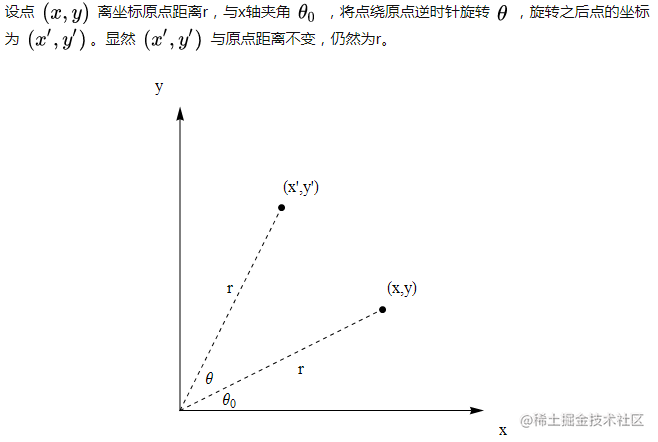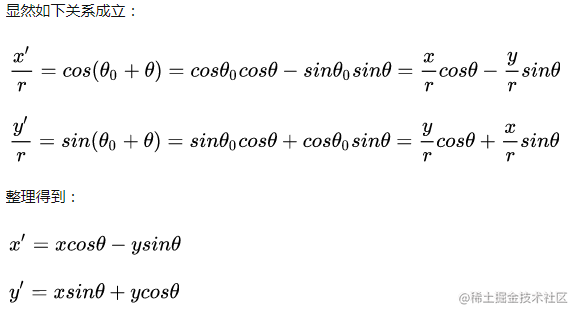``````function calculateLeftTop(style, curPositon, pointInfo) {
const { symmetricPoint } = pointInfo
const newCenterPoint = getCenterPoint(curPositon, symmetricPoint)
const newTopLeftPoint = calculateRotatedPointCoordinate(curPositon, newCenterPoint, -style.rotate)
const newBottomRightPoint = calculateRotatedPointCoordinate(symmetricPoint, newCenterPoint, -style.rotate)

const newWidth = newBottomRightPoint.x - newTopLeftPoint.x
const newHeight = newBottomRightPoint.y - newTopLeftPoint.y
if (newWidth > 0 && newHeight > 0) {
style.width = Math.round(newWidth)
style.height = Math.round(newHeight)
style.left = Math.round(newTopLeftPoint.x)
style.top = Math.round(newTopLeftPoint.y)
}
}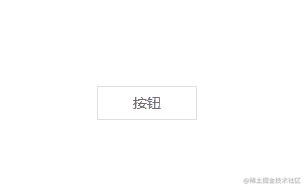### 自动吸附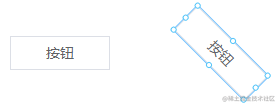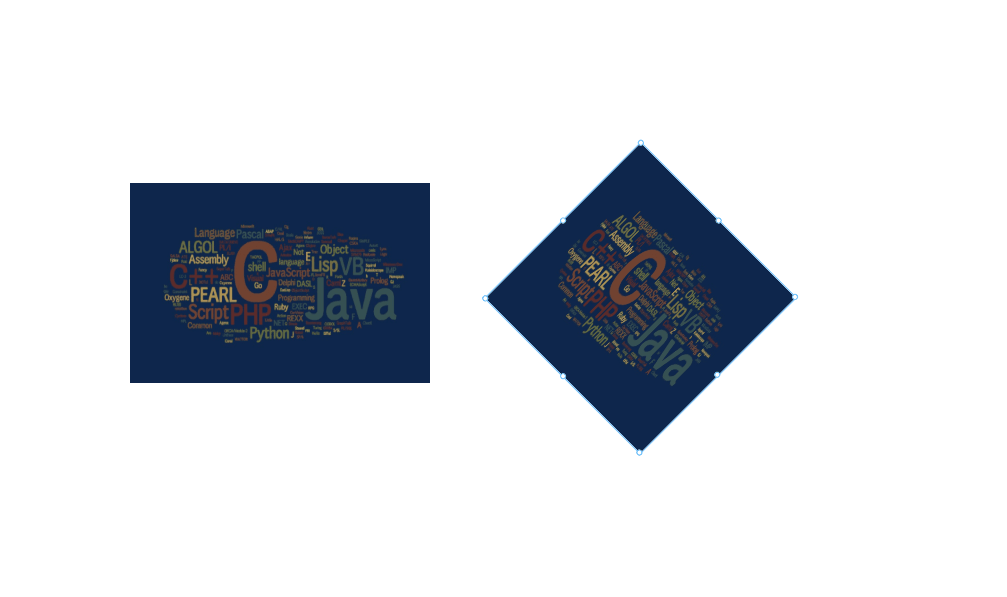#### 解决方案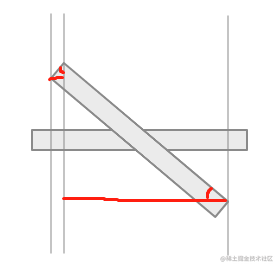``````const newWidth = style.width * cos(style.rotate) + style.height * sin(style.rotate)

``````const newHeight = style.height * cos(style.rotate) + style.width * sin(style.rotate)

``````translateComponentStyle(style) {
style = { ...style }
if (style.rotate != 0) {
const newWidth = style.width * cos(style.rotate) + style.height * sin(style.rotate)
const diffX = (style.width - newWidth) / 2
style.left += diffX
style.right = style.left + newWidth

const newHeight = style.height * cos(style.rotate) + style.width * sin(style.rotate)
const diffY = (newHeight - style.height) / 2
style.top -= diffY
style.bottom = style.top + newHeight

style.width = newWidth
style.height = newHeight
} else {
style.bottom = style.top + style.height
style.right = style.left + style.width
}

return style
}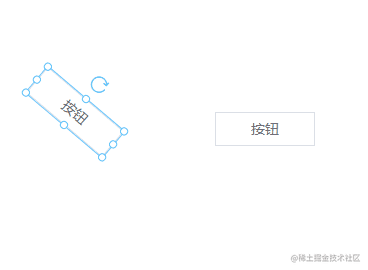### 光标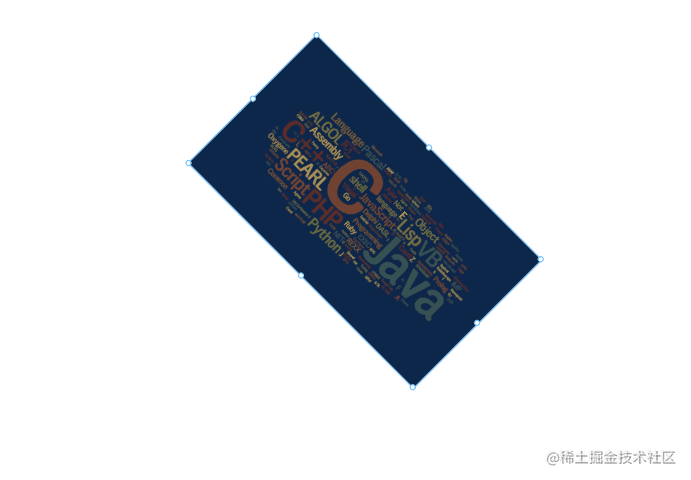#### 解决方案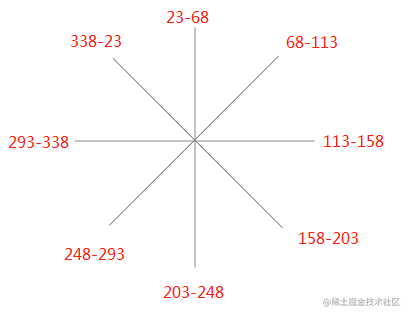``````pointList: ['lt', 't', 'rt', 'r', 'rb', 'b', 'lb', 'l'], // 八个方向
initialAngle: { // 每个点对应的初始角度
lt: 0,
t: 45,
rt: 90,
r: 135,
rb: 180,
b: 225,
lb: 270,
l: 315,
},
angleToCursor: [ // 每个范围的角度对应的光标
{ start: 338, end: 23, cursor: 'nw' },
{ start: 23, end: 68, cursor: 'n' },
{ start: 68, end: 113, cursor: 'ne' },
{ start: 113, end: 158, cursor: 'e' },
{ start: 158, end: 203, cursor: 'se' },
{ start: 203, end: 248, cursor: 's' },
{ start: 248, end: 293, cursor: 'sw' },
{ start: 293, end: 338, cursor: 'w' },
],
cursors: {},

1. 假设现在组件已旋转了一定的角度 a。
2. 遍历八个方向，用每个方向的初始角度 + a 得出现在的角度 b。
3. 遍历 `angleToCursor` 数组，看看 b 在哪一个范围中，然后将对应的光标返回。

``````getCursor() {
const { angleToCursor, initialAngle, pointList, curComponent } = this
const rotate = (curComponent.style.rotate + 360) % 360 // 防止角度有负数，所以 + 360
const result = {}
let lastMatchIndex = -1 // 从上一个命中的角度的索引开始匹配下一个，降低时间复杂度
pointList.forEach(point => {
const angle = (initialAngle[point] + rotate) % 360
const len = angleToCursor.length
while (true) {
lastMatchIndex = (lastMatchIndex + 1) % len
const angleLimit = angleToCursor[lastMatchIndex]
if (angle < 23 || angle >= 338) {
result[point] = 'nw-resize'
return
}

if (angleLimit.start <= angle && angle < angleLimit.end) {
result[point] = angleLimit.cursor + '-resize'
return
}
}
})

return result
},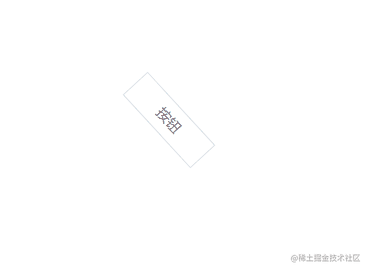## 15. 复制粘贴剪切

``````const ctrlKey = 17, vKey = 86, cKey = 67, xKey = 88
let isCtrlDown = false

window.onkeydown = (e) => {
if (e.keyCode == ctrlKey) {
isCtrlDown = true
} else if (isCtrlDown && e.keyCode == cKey) {
this.\$store.commit('copy')
} else if (isCtrlDown && e.keyCode == vKey) {
this.\$store.commit('paste')
} else if (isCtrlDown && e.keyCode == xKey) {
this.\$store.commit('cut')
}
}

window.onkeyup = (e) => {
if (e.keyCode == ctrlKey) {
isCtrlDown = false
}
}

### 复制操作

``````copy(state) {
state.copyData = {
data: deepCopy(state.curComponent),
index: state.curComponentIndex,
}
},

### 粘贴操作

``````paste(state, isMouse) {
if (!state.copyData) {
toast('请选择组件')
return
}

const data = state.copyData.data

if (isMouse) {
} else {
data.style.top += 10
data.style.left += 10
}

data.id = generateID()
store.commit('recordSnapshot')
state.copyData = null
},

### 剪切操作

``````cut(state) {
if (!state.curComponent) {
toast('请选择组件')
return
}

if (state.copyData) {
store.commit('addComponent', { component: state.copyData.data, index: state.copyData.index })
if (state.curComponentIndex >= state.copyData.index) {
// 如果当前组件索引大于等于插入索引，需要加一，因为当前组件往后移了一位
state.curComponentIndex++
}
}

store.commit('copy')
store.commit('deleteComponent')
},

### 右键操作

``````<li @click="copy" v-show="curComponent">复制</li>
<li @click="paste">粘贴</li>
<li @click="cut" v-show="curComponent">剪切</li>

cut() {
this.\$store.commit('cut')
},

copy() {
this.\$store.commit('copy')
},

paste() {
this.\$store.commit('paste', true)
},

## 16. 数据交互

### 方式一

``````<template>
<div>{{ propValue.data }}</div>
</template>

<script>
export default {
// propValue: {
//     api: {
//             request: a,
//             params,
//      },
//     data: null
// }
props: {
propValue: {
type: Object,
default: () => {},
},
},
created() {
this.propValue.api.request(this.propValue.api.params).then(res => {
this.propValue.data = res.data
})
},
}
</script>

### 方式二

``````const data = ['status', 'text'...]

``````// 组件能接收的数据
props: {
propValue: {
type: String,
},
element: {
type: Object,
},
wsKey: {
type: String,
default: '',
},
},

``````<template>
<div>{{ wsData[wsKey] }}</div>
</template>

<script>
import { mapState } from 'vuex'

export default {
props: {
propValue: {
type: String,
},
element: {
type: Object,
},
wsKey: {
type: String,
default: '',
},
},
computed: mapState([
'wsData',
]),
</script>

## 17. 发布

``````www.test.com/?id=a
www.test.com/?id=c
www.test.com/?id=b

### 按需加载

``````import Vue from 'vue'

const components = [
'Picture',
'VText',
'VButton',
]

components.forEach(key => {
Vue.component(key, () => import(`@/custom-component/\${key}`))
})

### 按版本发布

``````- v-text
- v1.vue
- v2.vue

``````{
component: 'v-text',
version: 'v1'
...
}

``````import Vue from 'vue'
import componentList from '@/custom-component/component-list`

componentList.forEach(component => {
Vue.component(component.name, () => import(`@/custom-component/\${component.name}/\${component.version}`))
})